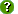# Dataset Details

Data Set ID: primet_230_a_5min_2020
Originator: Christopher Daly
Title: Meteorological data from Primary Met Station at the Andrews Forest. Variables include: battery supply, barometric pressure, swe, soil temperature, incoming shortwave radiation, precipitation, soil moisture from 01-Oct-2019 to 01-Oct-2020
Abstract: A three-level hydro-climatological network for data monitoring was established in 1994. The networks at each level are nested to form a coordinated program of data acquisition and measurement. A future vision of linking the benchmark meteorological stations with regional weather stations to expand the future scope of studies was also considered in designing this network. The first-level in this top-down approach consists of Benchmark Meteorological Stations (BMS) and Benchmark Stream Stations. The BMS are designed to represent the environment across the Andrews. These stations are intended to provide complete, long-term, high temporal resolution, meso-scale hydroclimatological data. The location of the BMS network is based on factors such as elevation, aspect, vegetation gradients, and accessibility. Collected meteorological parameters are generally standardized across the BMS as well as methods and instrumentation. Secondary Meteorological Stations also follow standardized methods and serve similar purposes but are somewhat limited in meteorological parameters collected. The Primary Meteorological Station (PRIMET), Central Meteorological Station (CENMET), Upper Lookout Meteorological Station (UPLMET), and Vanilla Leaf Meteorological Station (VANMET) are the four Benchmark Stations, Climatic Station at Watershed 2 (CS2MET) and the Hi-15 Meteorological Station (H15MET) are Secondary Stations.
Key Words: Air temperature, Climate change, Climate data, Meteorological measurements, Monitoring, Photosynthetically active radiation, Precipitation, Relative humidity, Snow accumulation, Snow hydrology, Soil moisture, Soil temperature, Solar radiation, Wind speed, Disturbance
Study Type: Monitoring
Study Period: 01-Oct-2019 to 01-Oct-2020
Bounding Box: West longitude:  unspecified
North latitude:  unspecified
East longitude:  unspecified
South latitude:  unspecified
Site References: PRIMET_230
Downloads:Data Table:  primet_230_a_5min_2020 (Main data table, 105409 records)

Access:  Public

Metadata:  Text (ESA FLED)

Data Files:  Spreadsheet (CSV Text) [21633kb], GCE Data Toolbox File [2829kb], Web Table (HTML text) [456kb]

Column List:

Column Name Units Type Description (show)
1 Site string Site code
2 Date serial number floating-point TmStamp
3 RecNum count integer RecNum
4 LOGGERID none integer LOGGERID
5 PROGID none floating-point Program ID of program
6 SOILTEMP_MEAN_0_10_01 deg c floating-point Average soil temperature
7 Flag_SOILTEMP_MEAN_0_10_01 none string QA/QC flags for Average soil temperature (flagging criteria, where "x" is SOILTEMP_MEAN_0_10_01: x<-2="I", x>35="I", x<-1="Q", x>30="Q", isnan(x)="M")
8 SOILTEMP_MEAN_0_20_02 deg c floating-point Average soil temperature
9 Flag_SOILTEMP_MEAN_0_20_02 none string QA/QC flags for Average soil temperature (flagging criteria, where "x" is SOILTEMP_MEAN_0_20_02: x<-2="I", x>35="I", x<-1="Q", x>30="Q", isnan(x)="M")
10 SOILTEMP_MEAN_0_50_03 deg c floating-point Average soil temperature
11 Flag_SOILTEMP_MEAN_0_50_03 none string QA/QC flags for Average soil temperature (flagging criteria, where "x" is SOILTEMP_MEAN_0_50_03: x<-2="I", x>35="I", x<-1="Q", x>30="Q", isnan(x)="M")
12 SOILTEMP_MEAN_0_100_04 deg c floating-point Average soil temperature
13 Flag_SOILTEMP_MEAN_0_100_04 none string QA/QC flags for Average soil temperature (flagging criteria, where "x" is SOILTEMP_MEAN_0_100_04: x<-2="I", x>35="I", x<-1="Q", x>30="Q", isnan(x)="M")
14 SOILWC_MEAN_0_10_01 fraction floating-point Average soil water content
15 Flag_SOILWC_MEAN_0_10_01 none string QA/QC flags for Average soil water content (flagging criteria, where "x" is SOILWC_MEAN_0_10_01: isnan(x)="M", x<0="I", x>.5="Q", x>1="I")
16 SOILWC_MEAN_0_20_02 fraction floating-point Average soil water content
17 Flag_SOILWC_MEAN_0_20_02 none string QA/QC flags for Average soil water content (flagging criteria, where "x" is SOILWC_MEAN_0_20_02: isnan(x)="M", x<0="I", x>.5="Q", x>1="I")
18 SOILWC_MEAN_0_50_03 fraction floating-point Average soil water content
19 Flag_SOILWC_MEAN_0_50_03 none string QA/QC flags for Average soil water content (flagging criteria, where "x" is SOILWC_MEAN_0_50_03: isnan(x)="M", x<0="I", x>.5="Q", x>1="I")
20 SOILWC_MEAN_0_100_04 fraction floating-point Average soil water content
21 Flag_SOILWC_MEAN_0_100_04 none string QA/QC flags for Average soil water content (flagging criteria, where "x" is SOILWC_MEAN_0_100_04: isnan(x)="M", x<0="I", x>.5="Q", x>1="I")
22 SOLAR_TOT_100_0_01 MJ floating-point Incoming shortwave radiation measured by Eppley Pyronameter
23 Flag_SOLAR_TOT_100_0_01 none string QA/QC flags for Incoming shortwave radiation measured by Eppley Pyronameter (flagging criteria, where "x" is SOLAR_TOT_100_0_01: isnan(SOLAR_MEAN_100_0_01)="M", isnan(x)="M", manual)
24 ATMPRESS_INST_0_0_01 mb floating-point Insantenous barometric pressure adjusted to sea level.
25 Flag_ATMPRESS_INST_0_0_01 none string QA/QC flags for Insantenous barometric pressure adjusted to sea level. (flagging criteria, where "x" is ATMPRESS_INST_0_0_01: x<800="Q", x>1200="Q", x<-2000="I", x>2000="I", isnan(x)="M")
26 SOLAR_MEAN_100_0_01 W m-2 floating-point Incoming solar radiation
27 Flag_SOLAR_MEAN_100_0_01 none string QA/QC flags for Incoming solar radiation (flagging criteria, where "x" is SOLAR_MEAN_100_0_01: x<=-10="I", x>1367="I", x>1060="Q", isnan(x)="M", Date>=datenum("02/18/2018 08:00:00")&Date<=datenum("02/22/2018 10:20:00")="T", , )
28 SOLAR_MAX_100_0_01 W m-2 floating-point Incoming maximum solar radiation
29 Flag_SOLAR_MAX_100_0_01 none string QA/QC flags for Incoming maximum solar radiation (flagging criteria, where "x" is SOLAR_MAX_100_0_01: )
30 PRECIP_TOT_100_0_01 mm floating-point Precipitation collected from the TE525 6in rain gauge
31 Flag_PRECIP_TOT_100_0_01 none string QA/QC flags for Precipitation collected from the TE525 6in rain gauge (flagging criteria, where "x" is PRECIP_TOT_100_0_01: x<0="I", x>10.16="I", isnan(x)="M", Date>=datenum("09/07/2019 00:05:00")&Date<=datenum("09/09/2019 12:00:00")="M")
32 PRECIP_ACC_100_0_01 mm floating-point Cumulative sum of total precipitation
33 Flag_PRECIP_ACC_100_0_01 none string QA/QC flags for Cumulative sum of total precipitation (flagging criteria, where "x" is PRECIP_ACC_100_0_01: )
34 SWE_INST_0_0_01 mm floating-point SWE_INST
35 Flag_SWE_INST_0_0_01 none string QA/QC flags for SWE_INST (flagging criteria, where "x" is SWE_INST_0_0_01: isnan(x)="M")
36 SWE_MED_0_0_01 mm floating-point 12 point running median of SWE_INST
37 Flag_SWE_MED_0_0_01 none string QA/QC flags for 12 point running median of SWE_INST (flagging criteria, where "x" is SWE_MED_0_0_01: )
38 BATTERY_AVG volts floating-point Battery supply voltage
39 Flag_BATTERY_AVG none string QA/QC flags for Battery supply voltage (flagging criteria, where "x" is BATTERY_AVG: x<0="I", x<11.5="L", x>15="H", isnan(x)="M", x>48="Q")
40 PAR_MEAN_100_0_01 umol/m2/s floating-point PAR calculated from SOLAR_MEAN_100_0_01. Total Solar (w/m2) = PAR 2.1 (umol/m2/s)
41 Flag_PAR_MEAN_100_0_01 none string QA/QC flags for PAR calculated from SOLAR_MEAN_100_0_01. Total Solar (w/m2) = PAR 2.1 (umol/m2/s) (flagging criteria, where "x" is PAR_MEAN_100_0_01: manual)
42 SOLAR_TOT_100_0_02 MJ/m2 floating-point Calculated total radiative energy. MJ/m2 = val_in_watts * number_seconds_in_period / 1000000
43 Flag_SOLAR_TOT_100_0_02 none string QA/QC flags for Calculated total radiative energy. MJ/m2 = val_in_watts * number_seconds_in_period / 1000000 (flagging criteria, where "x" is SOLAR_TOT_100_0_02: manual)
Last Modified: 06-May-2021 18:23:42